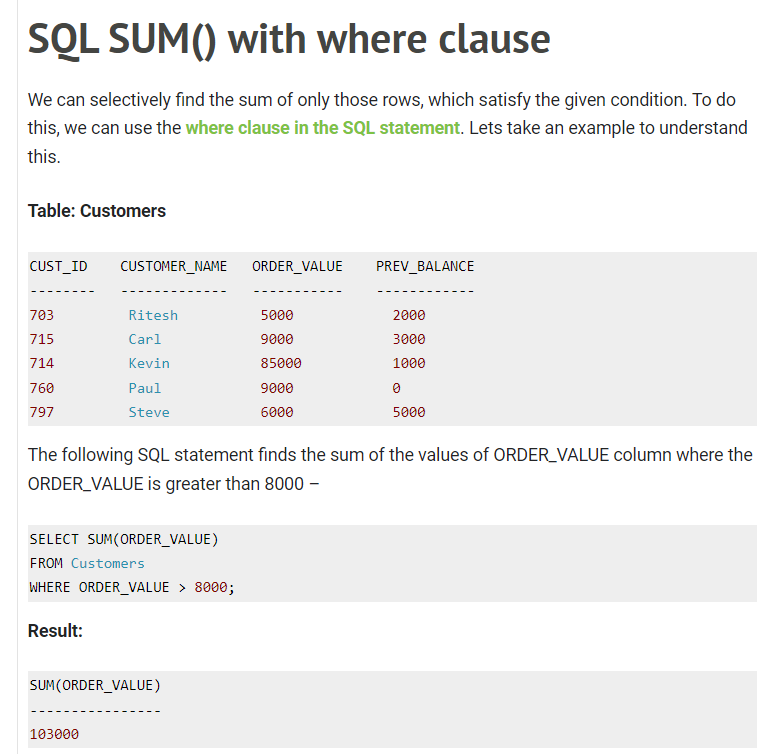# SUM() subquery in select clause

SUM 最重要還是 where clause select + where = filter select + sum() + where = satisfy 以前細個學又話叫 filter … 其實我覺得叫 satisfy 好d

select a, b, (select sum(xxx) where [CONDITION] 這裡放 group by 無用) as yyy, …

select …(select sum(xxx) where sth)只能回一個 single row , 唔係就會 error, 所以個 where clause 要俾夠 info 去砌到得翻一個 rowgroup by 本身 for multiple row 去教 table 點 group … 但 single row 其實就無必要

ref:# subquery is 帶出面既野入去 再收細個 dataset

https://stackoverflow.com/questions/20557899/how-does-subquery-in-select-statement-work-in-oracle

search more by keyword: Subqueries in SELECT Statements

# sql is relational algebra than a language

https://en.wikipedia.org/wiki/Relational_algebra